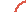Community MemberPosted: Tue Feb 10, 2009 @ 01:18am 0 commentsI've gotten addicted to writing in gibberish!!! >.<So I'm going to do a whole dictionary-ish-thing!!!q=œQ=Œw=∑W=„e=´E=´r=®R=‰t=†T=ˇy=¥Y=Áu=¨U=¨i=ˆI=ˆo=øO=Øp=πP=∏a=åA=Ås=ßS=Íd=∂D=Îf=ƒF=Ïg=©G=˝h=˙H=Ój=∆J=Ôk=˚K=l=¬L=Òz=ΩZ=¸x=≈X=˛c=çC=Çv=√V=◊b=∫B=ın=˜N=˜m=µM=Â`=`~=`1=¡!=⁄2=™@=€3=£#=‹4=¢\$=›5=∞%=ﬁ6=§^=ﬂ7=¶&=‡8=•*=°9=ª(=·0=º)=‚[=“{=”]=‘}=’;=…:=Ú'=æ"=Æ,=≤<=¯.=≥>=˘/=÷?=¿Ok! I'm done! biggrin